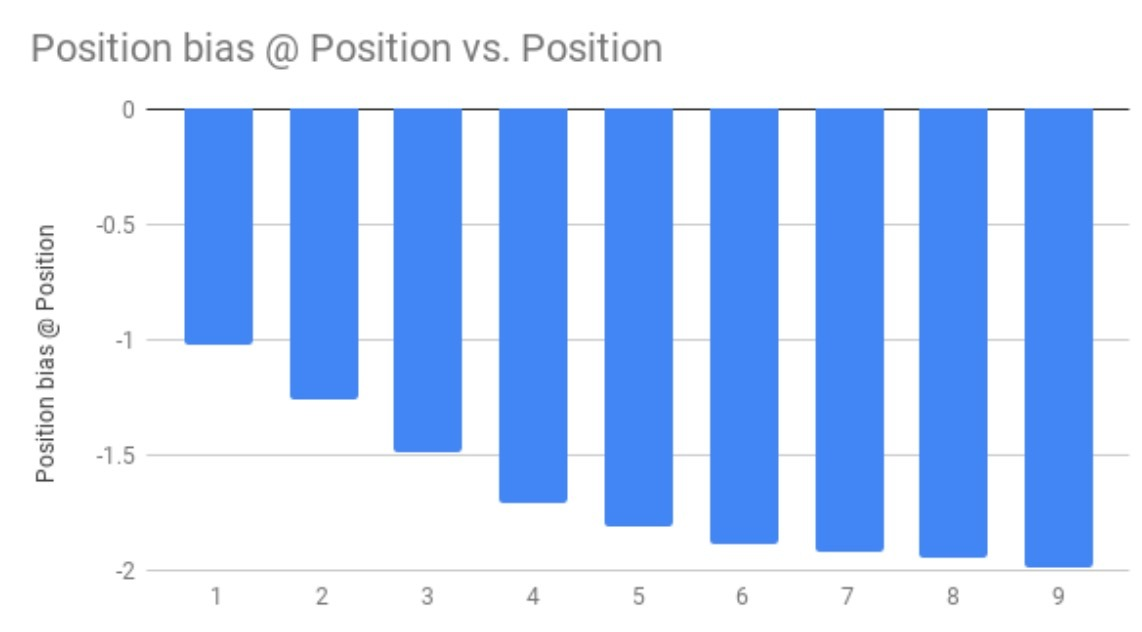# 介绍

facebook在2019在《Deep Learning Recommendation Model for Personalization and Recommendation Systems》。

# 1.介绍

• 1.使用embeddings来处理稀疏特征（sparse features）（它可以表示categorical data）
• 2.使用一个multilayer perceptron（MLP）来处理dense features

# 2.模型设计与架构

## 2.1 DLRM组件

### 2.1.1 Embeddings

$w_i^T = e_i^T W$

…(1)

$a^T = [0, \cdots, a_{i_1}, \cdots, a_{i_k}, \cdots, 0]$

• 对于$i=i_1, \cdots, i_k$，元素$a_i \neq 0$，否则为0 ，其中$i=i_1, \cdots, i_k$是相应的items。

$S = A^T W$

…(2)

DLRMs会使用embedding tables来将categorical features映射成dense representations。然而，在这些embeddings被设计后，如何利用它们来生成更精准的预测呢？我们先来回顾下latent factor。

### 2.1.2 Matrix Factorization

• $w_i \in R^d, i=1,\cdots, n$来表示第i个商品，
• $v_j \in R^d, j=1, \cdots, m$来表示第j个user

MF方法通过最小化下面的等式来求解该问题：

$min \sum\limits_{(i,j) \in S} r_{ij} - w_i^T v_j$

…(3)

• $r_{ij} \in R$是第j个user对第i个product的rating，$i=1, \cdots, m; j = 1, \cdots, n$。

### 2.1.3 Factorization Machine

FM使用categorical data，通过定义以下形式的模型，来将二阶交叉并入到一个线性模型中：

$\hat{y} = b + w^T x + x^T upper(VV^T) x$

…(4)

• 1.$V \in R^{n \times d}$
• 2.$w \in R^n$
• 3.$b \in R$
• 4.$d << n$的参数
• 5.upper会严格选择该矩阵的上三角部分【24】。

FM与SVM和polynomial kernels有明显区别，因为它们将二阶交叉矩阵分解成latent factors（或embedding vectors）（和MF很像），它能更有效地处理稀疏数据。通过只捕获不同embedding vectors pairs间的交叉，这可以极大减小二阶交叉的复杂度，生成线性的计算复杂度

### 2.1.4 MLP(Multilayer Perceptrons)

$\hat{y} = W_k \sigma(W_{k-1} \sigma(W_1 x + b_1) \cdots) + b_{k-1}) + b_k)$

…(5)

• $W_l \in R^{n_l \times n_{l-1}}$是weight matrix
• $b_l \in R^{n_l}$：表示对于$layer \ l=1,\cdots,k$的bias

## 2.2 DLRM架构

• 为了处理categorical features，每个categorical feature可以通过一个相同维度的embedding vector表示，即MF中latent factors。
• 为了处理continous features，会通过一个MLP来进行转换，它会生成和embedding vectors相同长度的dense representation## 2.3 与之前的模型比较

DLRM和其它网络之间的一个关键不同点是：这些网络是如何对待embedded feature vectors和它们的cross-terms的DLRM(以及XDeepFM)会将每个feature vector看成单个unit来表示单个category，其它像DCN(Deep&Cross)网络会将feature vector中的每个element看成是一个新的unit，这会生成不同的cross-terms。因此，Deep&Cross网络不仅会生成不同feature vectors的elements间的cross-terms（这和DLRM通过dot product方式一样），也会生成在相同feature vector的elements间的cross-terms，从而生成更高的维度。

# feedback loops介绍

deepmind在19年发了篇关于feedback loops的paper：《Degenerate Feedback Loops in Recommender Systems》，我们可以来看下它的paper介绍：

。。。

# 3.模型

• 在timestep t上，user在一个item $a \in M$上的兴趣，可以通过函数$\mu_t: M \rightarrow R$来描述。
• 如果用户对该item很感兴趣，那么$\mu_t(a)$很大（positive）；反之为很小（negative）• 如果用户在timestep t上对一个item a进行点击，那么$\mu_{t+1}(a) > \mu_t(a)$；
• 如果a被展示但未被点击，则$\mu_{t+1}(a) < \mu_t(a)$. （这里, $c_t \in \lbrace 0, 1 \rbrace^l$可以被定义成所对应items的关于点击的indicator vector）

$\underset{t \rightarrow \infty} {lim} sup || \mu_t - \mu_0 ||_2 = \infty,$

…(1)

$\underset{t \rightarrow \infty} {lim} || \mu_t - \mu_0 ||_2 = \infty$

…(2)

# 3. 用户兴趣动态性——Echo Chamber

$\mu_{t+1} = \mu_t + f(\mu_t, \xi_t)$

• 我们假设$\mu_0 \in R$是固定的
• $(\xi_t)_{t=1}^{\infty}$是一个关于独立均匀分布的随机变量的无限序列，它引入噪声到系统中（例如：$\mu_{t+1}$是一个$\mu_t$的随机函数）
• 函数 $f: R \times [0, 1]$是假设是可测的，但其它任意。通过$U([0, 1])$来定义[0,1]上的均匀分布，假设：
$\bar{f}(\mu) = E_{\xi \sim U([0,1])} [f(\mu, \xi)]$

$F(\mu, x) = P_{\xi \sim U([0,1])} (f(\mu, \xi) \leq x)$

• 1) 对于所有$\mu \geq \mu_o$，有$F(\mu, 0) < 1$
• 2) 对于所有$\mu \geq \mu_o$，有$F(\mu, 0) > 0$

# 4.系统设计角色——filter bubble

## 4.1 Model accuracy

$\mu_t(a) = (\mu_0(a) + \frac{b(a)}{k(a)} (1 + k(a))^t - \frac{b(a)}{k(a)}$

Sufacing Assuption:

# 5.模拟实验

$\mu_{t+1}(a_t^i) - \mu_t(a_t^i) = \begin{cases} \delta(a_t^i) & \text{if  c_t(a_t^i) = 1  } \\ -\delta(a_t^i) & \text{otherwise} \end{cases}$

…(3)

• Random model
• Oracle
• UCB Multi-armed bandit
• Thompson Multi-armed bandit

## 6.1 Echo Chamber & Filter Bubble effectDegenracy SpeedCandidate Pool SizeNoise Level的效应Growing Candidate Pool。。。

# 3.Popularity bias和miscalibration

## 3.1 Miscalibration

miscalibration用来measure用户真实偏好与推荐算法间差异程度。前面提到，如果在所有用户上都存在miscalibration，可能意味着个性化算法的failure。当不同user groups具有不同程度的miscalibration时，意味着对特特定user groups存在unfair treatment。

。。。

• $p_u(c \| u)$：在过去用户u评分过的items集合$\Gamma$上的feature c的分布为：
$p_u(c | u) = \frac{\sum\limits_{i \in \Gamma w_{u,i} p(c|i)}}{\sum\limits_{i \in \Gamma} w_{u,i}}$

…(1)

• $q_u(c \| u)$：推荐给user u的items list的feature c上的分布：
$q_u(c|u) = \frac{\sum\limits_{i \in \wedge} w_r(i) p(c|i)}{ \sum\limits_{i \in \wedge} w_r(i)}$

…(2)

$\wedge$表示推荐items集合。item i的weight则表示推荐中的rank r(i)。比如：MRR或nDCG。此外我们将$w_r$设置为1, 确保$q_u$和$p_u$可比。

$MC_u(p_u, q_u) = H(p_u, q_u) = \frac{|| \sqrt{p_u} - \sqrt{q_u}||_2}{\sqrt{2}}$

…(3)

$MC_G(p, q) = \frac{\sum_{u \in G} MC_u(p_u, q_u)}{|G|}$

….(4)

# 介绍

alibaba在《Personalized Re-ranking for Recommendation》介绍了一种reranking模型。

# 摘要

ranking是推荐系统的核心问题，通常，一个ranking函数会从labeled dataset中学到，并会为每个单独item产生一个ranking score。然而，它可能是次优的(sub-optimal)，因为scoring function被应用在每个独立item上，没有显式考虑item间的相互影响，以及用户偏好／意图间的不同。因此，这里提出了一种个性化的re-ranking模型。通过直接使用一个已经存在的ranking feature vectors，提出的re-ranking模型可以很轻易地部署成在任意ranking算法之后跟着的一个follow-up模块。它会通过采用一个transformer结构来对在推荐列表中的所有items信息进行有效编码，来直接优化整个推荐列表。特别的，transformer使用一种self-attention机制，它直接建模在整个list中任意items pair间的关系。我们证实，通过引入pre-trained embedding来为不同用户学习个性化编码函数。在offline和online的实验结果上均有较大提升。

# 2.相关工作

【1-3,37】的工作主要是re-ranking方法。它们使用整个intial list作为input，并以不同方式建模在items间的复杂依赖。使用unidirectional GRU来将整个list的信息编码到每个item的表示。使用LSTM、使用pointer network，不仅编码整个list信息，也会由decoder生成ranked list。对于这些使用GRU or LSTM的方法来编码items间的依赖，encoder的能力通过encoding distance进行限制。在我们的paper中，我们使用transfomer-like encoder，它基于self-attention机制以O(1) distance来建模任意两个items间的交叉。另外，对于那些使用decoder来顺序生成ordered list的方法，它们不适合online ranking系统，因为需要有严格的延迟。由于sequential decoder使用在time t-1上选中的item作为input来在time t上选择item，它不能并列行，需要n倍的inferences，其中n是output list的长度。提出了一种groupwise scoring function，它可以对scoring进行并列化，但它的计算开销很高，因为它会枚举在list中所有可能的item组合。

# 3.re-ranking模型l2r方法在ranking中被广泛使用，为推荐和信息检索生成一个ordered list。l2r方法会基于items的feature vector学习一个global scoring function。有了该global function，l2r方法会通过在candidate set中的每个item。该global function通常通过对以下loss L最小化得到：

$L = \sum\limits_{r \in R} l( \lbrace y_i, P(y_i | x_i;\theta) | i \in I_r \rbrace )$

…(1)

• R是对于推荐所有用户请求的集合。
• $I_r$是对于请求$r \in R$的items的candidate set
• $x_i$表示的是item i的feature space
• $y_i$是在item i上的label (例如：click or not)
• $P(y_i \mid x_i; \theta)$是由ranking model给出的对于参数$\theta$的预测点击概率
• l是通过$y_i$和$P(y_i \mid x_i; \theta)$计算得到的loss

• (a)item-pairs间的相互影响 [8,35]
• (b)users和items间的交叉(interactions)

$L = \sum\limits_{r \in R} l( \lbrace y_i, P(y_i \mid X, PV; \hat{\theta}) | i \in S_r \rbrace )$

…(2)

• $S_r$是由之前ranking model给出的inital list
• $\hat{\theta}$是我们的re-ranking model的参数
• X是在list中所有items的feature matrix

# 4.个性化re-ranking模型

## 4.1 模型架构

PRM的结构如图1所示。模型包含三个部分：

• input layer
• encoding layer
• output layer## 4.2 Input Layer

input layer的目的是，为在initial list中的所有items准备representations，并将它feed给encoding layer。首先，我们具有一个固定长度的intial sequential list $S=[i_1, i_2, \cdots, i_n]$，它由之前的ranking方法给出。与之前ranking方法相同，我们具有一个raw feature matrix $X \in R^{n \times d_{feature}}$，在X中的每一行表示每个$i \in S$的item对应的raw feature vector $x_i$。

Personalized Vector(PV)

$E' = \left[ \begin{array} x_{i_1}; pv_{i_1} \\ x_{i_2}; pv_{i_2} \\ \cdots \\ x_{i_n}; pv_{i_n} \end{array} \right]$

…(3)

position embedding(PE)

$E'' = \left[ \begin{array} x_{i_1}; pv_{i_1} \\ x_{i_2}; pv_{i_2} \\ \cdots \\ x_{i_n}; pv_{i_n} \end{array} \right] + \left[ \begin{array} pe_{i_1} \\ pe_{i_2} \\ \cdots \\ pe_{i_n} \end{array} \right]$

…(4)

$E = EW^E + b^E$

…(5)

## 4.3 Encoding Layer

Attention Layer

attention函数如等式(6)所示：

$Attention(Q,K,V) = softmax(\frac{QK^T}{\sqrt{d}})V$

…(6)

$S' = MH(E) = Concat(head_1, \cdots, head_h) W^O \\ head_i = Attention(EW^Q, EW^K, EW^V)$

…(7)

FFN(Feed-forward Network)

## 4.4 Output Layer

output layer的函数主要为每个item $i = i_1, \cdots, i_n$生成一个score。（如图1(b)所示Score(i)）我们在softmax layer之后使用一个linear layer。softmax layer的output是每个item的点击概率，被标记为：$P(y_i \mid X, PV; \hat{\theta})$。我们使用$P(y_i \mid X, PV, \hat{\theta})$作为$Score(i)$来在one-step中对items进行re-rank。Score(i)的公式为：

$Score(i) = P(y_i \mid X, PV; \hat{\theta}) = softmax(F^{(N_x)}W^F + b^F), i \in S_r$

…(8)

• $F^{(N_x)}$是Transformer encoder的$N_x$个blocks的output
• $W^F$是可学习的投影矩阵
• $b^F$是bias term
• n是在intial list中的items数目

$L = - \sum\limits_{r \in R} \sum\limits_{i \in S_r} y_i log(P(y_i | X, PV; \hat{\theta})$

…(9)

## 4.5 个性化模块

$L = \sum\limits_{i \in D} (y_i log( P(y_i | H_u, u; \theta')) + (1-y_i) log(1-P(y_i | H_u,u;\theta')$

…(10)

• D是user u在平台上展示的items set。
• $\theta'$是pre-trained model的参数矩阵
• $y_i$是item i的label

# 介绍

• 通常有许多不同的、有时甚至有冲突的待优化目标。例如，我们想推荐用户点击率高、愿与朋友共享的、包括观看高的视频
• 在该系统中通常有隐式偏差（implicit bias）。例如，一个用户通常点击和观看一个视频，仅仅只因为它的排序高，而不是因为用户最喜欢它。因此，从当前系统的数据生成来进行模型训练会是有偏的，这会造成(feedback loop effect)效应。如何有效和高效地学习减少这样的biases是个开放问题。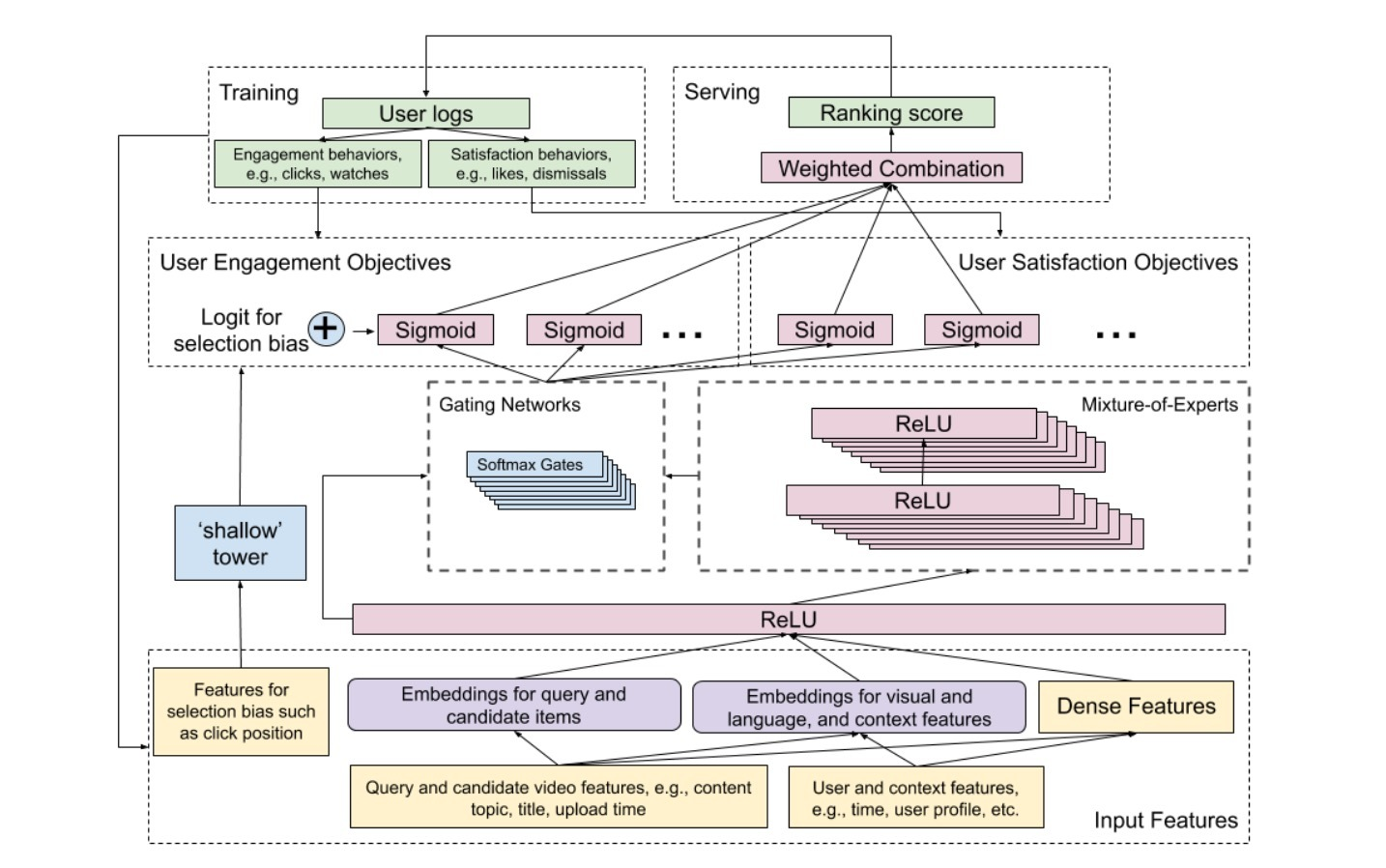• 1) 参与度目标(engagement objectives)，比如：用户点击(user clicks)，推荐视频的参与度
• 2) 满意度目标（satisfaction objectives），比如：用户喜欢一个视频的程度，在推荐上留下一个评分

• 1.从主模型中学到的无偏用户效用(unbiased user utility)
• 2.从shallow tower学到的估计倾向评分(estimated propensity score)

• 1) 多任务学习
• 2) 移除一个常见的selection bias (position bias)

• 介绍了一种end-to-end的排序系统来进行视频推荐
• 将ranking问题公式化成一个多目标学习问题，并扩展了Multi-gate Mixture-of-Experts架构来提升在所有objectives上的效果
• 我们提出使用一个Wide&Deep模型架构来建模和缓和position bias
• 我们会在一个真实世界的大规模视频推荐系统上评估我们的方法，以及相应的提升

# 3.问题描述

• 多模态特征空间(Multimodal feature space)。在一个context-aware个性化推荐系统中，我们需要使用从多模态（例如：视频内容、预览图、音频、标题、描述、用户demographics）来学习候选视频的user utility。从多模态特征空间中为推荐学习表示，对比其它机器学习应用来说是独一无二的挑战。它分为两个难点：
• 1) 桥接来自low-level的内容特征中的语义gap，以进行内容过滤(content filtering)
• 2) 为协同过滤学习items的稀疏表示
• 可扩展性（Scalability）。可扩展性相当重要，因为我们正构建一个数十亿用户和视频的推荐系统。模型必须在训练期间有效训练，在serving期间高效运行。尽管ranking system在每个query会对数百个candidates进行打分，真实世界场景的scoring需要实时完成，因为一些query和context信息不仅仅需要学习数十亿items和users的表示，而且需要在serving时高效运行。

# 4.模型结构

## 4.1 系统总览

• 1) engagement行为（比如：点击和观看）
• 2) satisfaction行为（比如：喜欢(likes)和dismissals）

## 4.2 ranking objectives

Engagement objectives会捕获user behaviors（比如：clicks和watches）。我们将这些行为的预测公式化为两种类型的任务：对于像点击这样行为的二元分类任务，以及对于像时长(time spent)相关的行为的回归任务。相似的，对于satisfaction objectives，我们将：与用户满意度相关的行为预测表示成二元分类任务或者回归任务。例如，像点击/like这样的行为可以公式化成一个二元分类任务，而像rating这样的行为被公式化成regression任务。对于二元分类任务，我们会计算cross entropy loss。而对于regression任务，我们会计算squared loss。

## 4.3 使用MMoE建模任务关系和冲突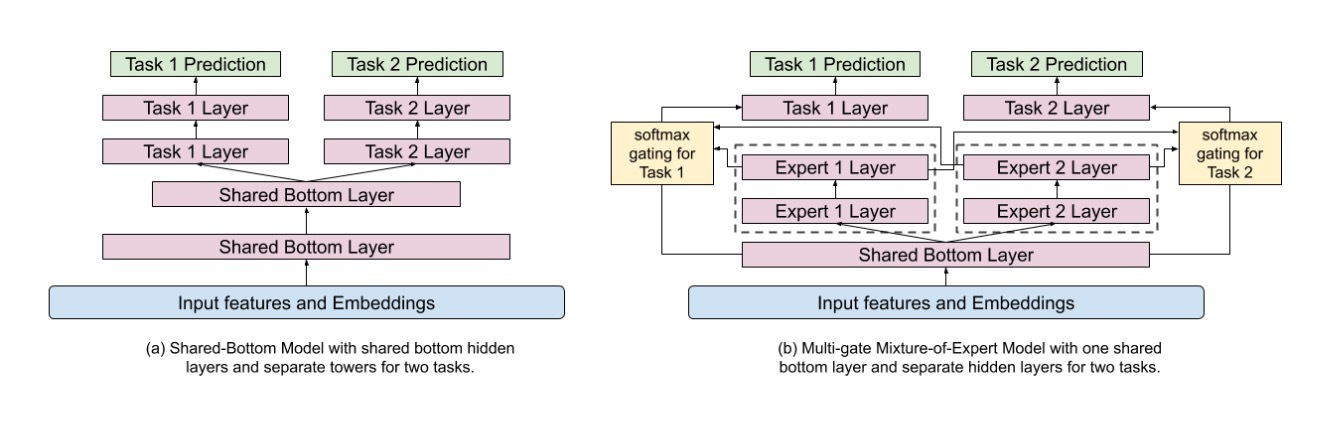$y_k = h^k (f^k(x)), \\ where \ \ f^k(x) = \sum\limits_{i=1}^n g_{(i)}^k(x) f_i(x)$

…(1)

• $x \in R^d$是一个lower-level shared hidden embedding
• $g^k$是task k的gating network
• $g_{(i)}^k(x) \in R^n$是第i个entry
• $f_i(x)$是第i个expert

gating networks是使用一个softmax layer的关于input的简单线性转换。

$g^k(x) = softmax(W_{g^k} x)$

…(2)

$W_{g^k} \in R^{n \times d}$是线性变换的自由参数

## 4.4 建模和移除Position和Selection Baises

• 来自main tower的一个user-utility component
• 以及来自shallow tower的一个bias component

• 在训练中，所有曝光（impressions）的positions都会被使用，有10%的feature drop-out rate来阻止模型过度依赖于position feature
• 在serving时，position feature被认为是缺失的(missing)。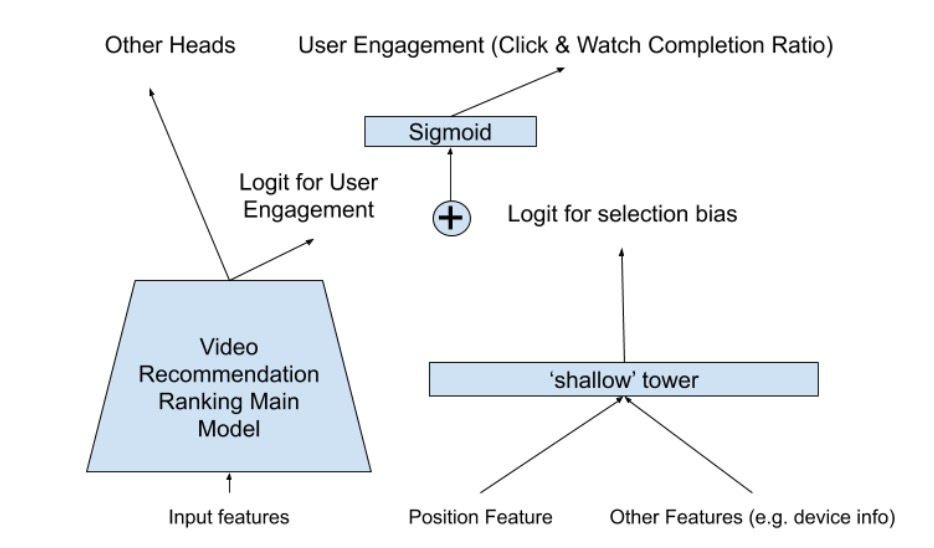# 5.实验结果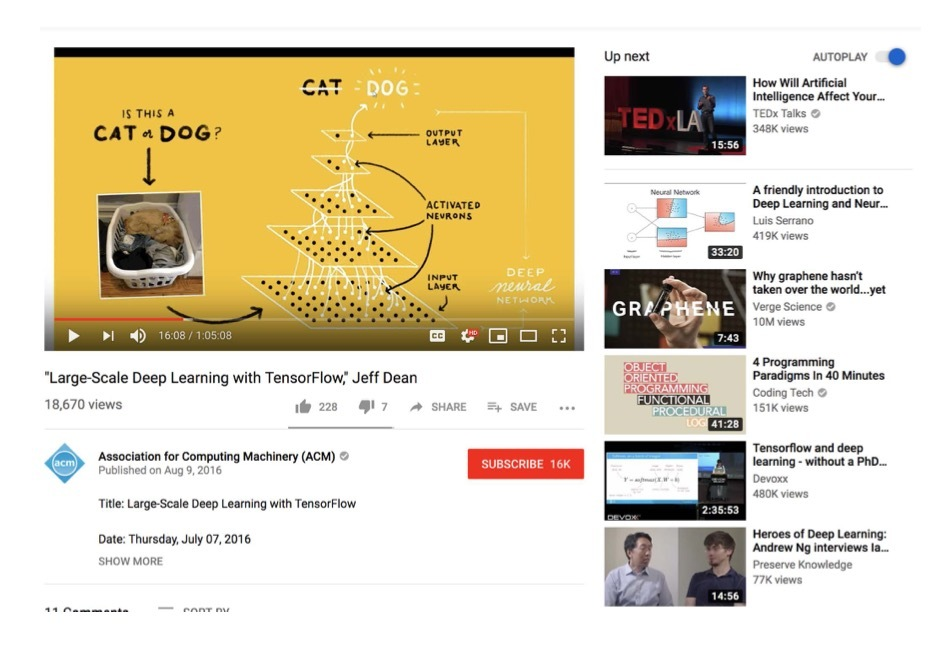## 5.3 建模和减小Position Bias

### 5.3.1 用户隐反馈分析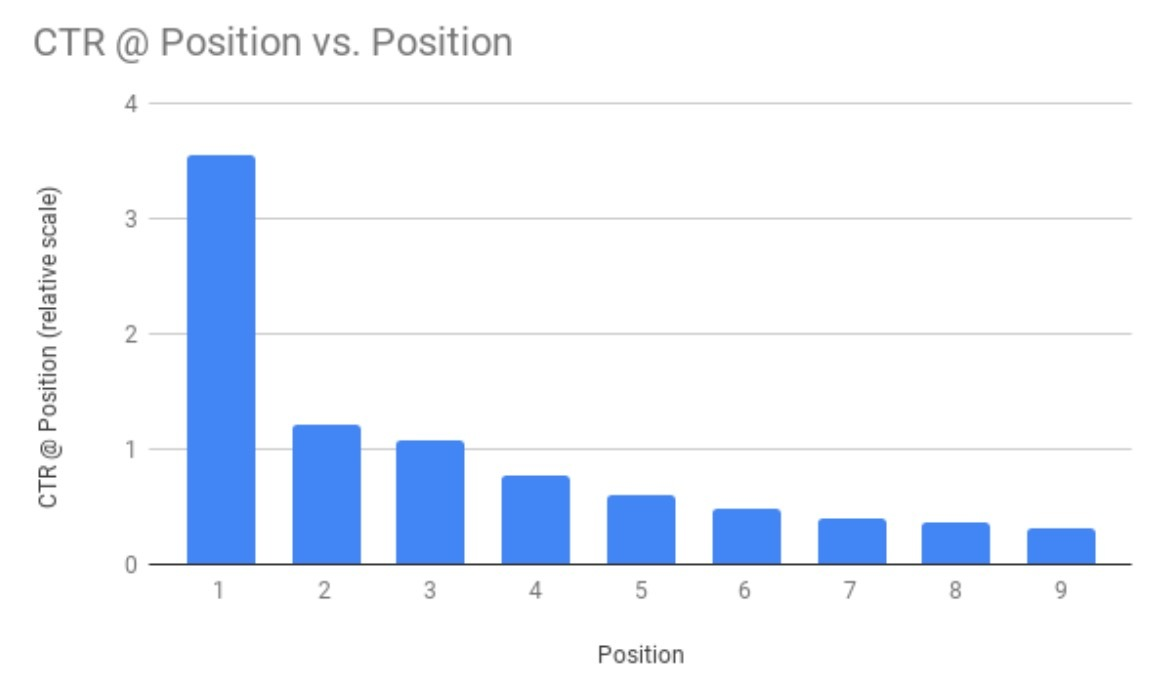### 5.3.2 Baseline方法

• 直接使用position feature做为一个input feature：这种简单方法已经在工业界推荐系统中广泛使用来消除position bias，大多数用于线性l2r rank模型中。

### 5.3.4 学到的position biases